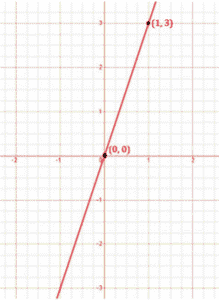Newbie

# Draw the graph of each of the following linear equations in two variables:(iii) y=3x . Q.1(3)

• 0

Today I am solving the ncert class 9th solution of chapter linear equation in two variables .Find the best solution of exercise 4.3 question number 1(3), also find the simplest and easiest solution of this question. Draw the graph of each of the following linear equations in two variables:(iii) y=3x .

Share

1. Solution:

To draw a graph of linear equations in two variables, let us find out the points to plot.

To find out the points, we have to find the values which x and y can have, satisfying the equation.

Here,

y = 3x

Substituting the values for x,

When x = 0,

y = 3x

y = 3×0

y = 0

When x = 1,

y = 3x

y = 3×1

y = 3

 x y 0 0 1 3

The points to be plotted are (0, 0) and (1, 3)• 0